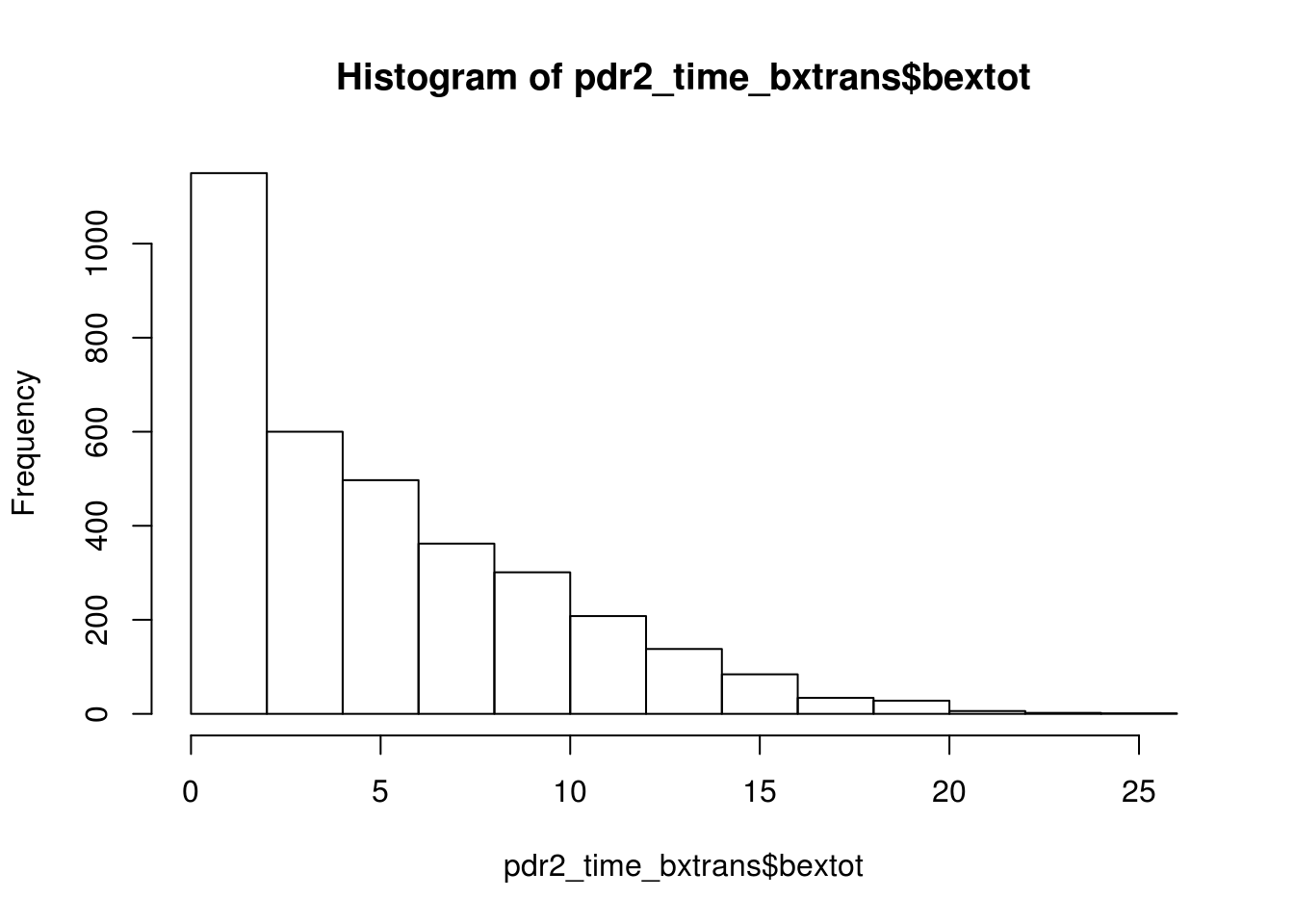# Latent Growth Curves in R

This is based in part on Nicole’s code found in this post.

sem_in_r.r

``````library(foreign)
library(dplyr)
library(tidyr)
library(ggplot2)
library(lavaan)
library(semPlot)
library(knitr)

setwd('/home/jflournoy/code/sem_in_r/')

## Generate a time variable

This indexes each call for each family.

``````pdr2_time <- pdr2 %>%
group_by(FAMILY) %>% #do the count by family
arrange(YEAR,MONTH,DAY) %>% #sort by date
mutate(callindex=1:n()) #create call index that's 1:end for each family

``````## Source: local data frame [6 x 2]
## Groups: FAMILY
##
##   FAMILY callindex
## 1  TP001         1
## 2  TP001         2
## 3  TP001         3
## 4  TP001         4
## 5  TP001         5
## 6  TP001         6``````

## Create composite score of kid bex

``````pdr2_time_bxtrans<-pdr2_time %>% ungroup %>%
mutate_each(
funs(as.numeric(!(.=='DID NOT OCCUR'))),
P31201:P31240)``````

To break down the above statement:

`pdr2_time` gets sent to ungroup, which removes the grouping by `FAMILY` we did above, and that gets sent to `mutate_each`. This takes a range of columns that we define in the second argument as `P31201:P31240` which reads ‘from P31201 to P31240’.

The meat of `mutate_each` is what goes in the first argument, within the function `funs()`. You can list a bunch of functions here if you wanted to mutate all the columns in a number of different ways. The period character, `.`, represents the column that will be passed to that function. In this case, we just check if each element of the column is ‘DID NOT OCCUR’, and if so, we negate it (giving us `FALSE`) and then `as.numeric` it giving us ‘0’. If the response is any other option, we get a ‘1’, which is what we want. Importantly, this will return `NA` if the data is `NA`.

If you want to learn more `?mutate_each`. Moving on now…

``head(pdr2_time_bxtrans)``
``````## Source: local data frame [6 x 63]
##
##   FAMILY RESP MONTH DAY YEAR INT   WEEKDAY P31201 P31202 P31203 P31204
## 1  TP001    3     7  22 2004  4H WEDNESDAY      0      1      1      0
## 2  TP001    3     7  23 2004  4H   THURSAY      0      1      1      0
## 3  TP001    3     7  27 2004  4H    MONDAY      0      1      1      0
## 4  TP001    3     7  28 2004  4H   TUESDAY      0      1      1      0
## 5  TP001    3     8   3 2004  4H    MONDAY      0      1      1      0
## 6  TP001    3     8   4 2004  4H   TUESDAY      0      1      1      0
## Variables not shown: P31205 (dbl), P31206 (dbl), P31207 (dbl), P31208
##   (dbl), P31209 (dbl), P31210 (dbl), P31211 (dbl), P31212 (dbl), P31213
##   (dbl), P31214 (dbl), P31215 (dbl), P31216 (dbl), P31217 (dbl), P31218
##   (dbl), P31219 (dbl), P31220 (dbl), P31221 (dbl), P31222 (dbl), P31223
##   (dbl), P31224 (dbl), P31225 (dbl), P31226 (dbl), P31227 (dbl), P31228
##   (dbl), P31229 (dbl), P31230 (dbl), P31231 (dbl), P31232 (dbl), P31233
##   (dbl), P31234 (dbl), P31235 (dbl), P31236 (dbl), P31237 (dbl), P31238
##   (dbl), P31239 (dbl), P31240 (dbl), P31241 (fctr), P31242 (fctr), P31242A
##   (fctr), P31242B (fctr), P31242C (fctr), P31242D (fctr), P31243 (fctr),
##   P31243A (fctr), P31243B (fctr), P31243C (fctr), P31243D (fctr), P31244
##   (dbl), P31245 (dbl), WAVE (dbl), PILOT1 (fctr), callindex (int)``````

Now we can create the composite variable using a sum. There is missing data, so that should be delt with, but we’ll ignore that for now.

``````pdr2_time_bxtrans\$bextot<-pdr2_time_bxtrans %>%
select(P31201:P31240) %>% rowSums(na.rm=T)

summary(pdr2_time_bxtrans\$bextot)``````
``````##    Min. 1st Qu.  Median    Mean 3rd Qu.    Max.
##   0.000   1.000   4.000   5.307   8.000  26.000``````
``hist(pdr2_time_bxtrans\$bextot)``We have a call-index varibale, but if you want a time index that is really the number of days since they were first contacted, here’s how to do that.

``````pdr2_time_bxtrans_t2<-
pdr2_time_bxtrans %>%
mutate(formd_date=as.Date(paste(MONTH,DAY,YEAR,sep='/'),"%m/%d/%Y")) %>%
group_by(FAMILY) %>%
arrange(YEAR,MONTH,DAY) %>%
mutate(days_since_1st=formd_date-min(formd_date))``````

## Some descriptive plots

Let’s see what we’re working with.

First, the raw data – a line for every family:

``````pdr2_time_bxtrans_t2 %>% filter(callindex < 33) %>%
ggplot(aes(x=callindex,y=bextot))+
geom_line(aes(group=FAMILY),alpha=.1)+
theme(panel.background=element_rect(fill='white'))``````